## Python Tutorials: Python Plotting with Matplotlib

##### Python Tutorials: In this part of Learning Python we Cover Plotting Graph with Matplotlib Python
Written by |17-Oct-2020 | 1 Comments | 1133 Views

Legends:

In Matplotlib, we used legends to indicate a different type of dataset. Suppose a naive person or a person who doesn’t know how to code. If he or she sees the plot, he will never understand which line indicate what sort of data. In such a scenario’s legends come. Legends are the labels of a specific data set. It shows not only the label but also the type of line by which it is to identify the data set.

Let’s take the example from the previous section and apply the legends to differentiate the data set visually.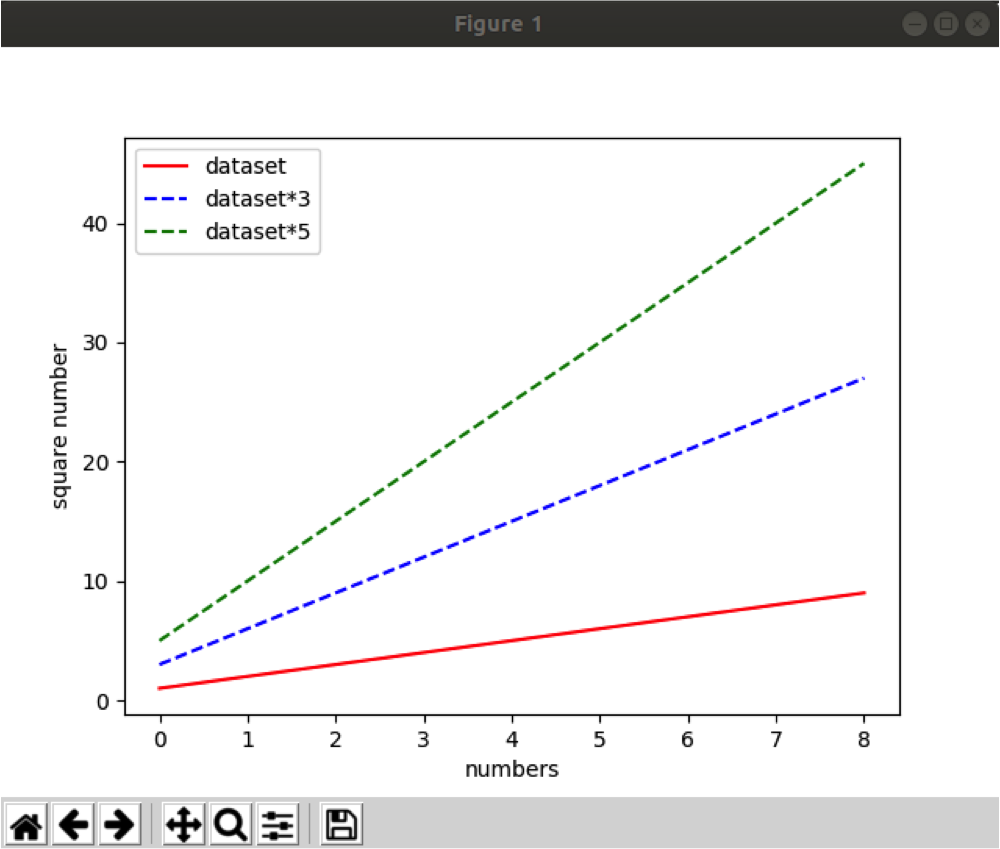Figure 1

Now we know that the red line for the original dataset, blue for dataset*3, and green for dataset*5.

To add the legend and labels in a figure, we have to add the additional argument, which is the label, and assign the string value to it. The label will not show in the figure until we will call the legend function. Plt.legend() will display the labels that we assigned in the plot function as an argument, as can be seen in the following code.

 import matplotlib.pyplot as plt data = [1,2,3,4,5,6,7,8,9] plt.plot(data,'r-', label='dataset') plt.plot([i * 3 for i in data],'b--', label='dataset*3') plt.plot([i * 5 for i in data],'g--', label='dataset*5') plt.ylabel('square number') plt.xlabel('numbers') plt.legend() plt.show()

Subplots:

As we have seen, we can plot multiple datasets in the same window. But that data set was related to each other what if we want to plot two different data sets and we want to plot on the same window but not overlapping each other. In this scenario, we will use a subplot. Subplot allows us to make multiple windows in the main root window. The data set will not overlap with each other and will be outputted as individual data.

Following is the code of plotting a subplot:

 import matplotlib.pyplot as plt data1 = [0.5, 0.5, 1, 1, 0, 0, 1, 1, 0, 0, 1, 1, 0, 0.5, 0.5] data2 = [1,2,3,4,5,6,7,8,9] plt.figure(1) plt.subplot(211) plt.plot(data1, 'b-') plt.subplot(212) plt.plot(data2, 'r-') plt.show()

What the code mentioned above does it will first import the Matplotlib module and then make a random data set.

After that, we have initiated the instance of the figure. The figure is the window in which we want to plot the subplots. After initiating the figure, we initialize the subplot. It is compulsory to give the 3-digit integer of which 1st two digits must be twenty-one. And then we plot the data 1. The same code is applied to data 2, and in last, we show the graph. The plotted graph looks as follows: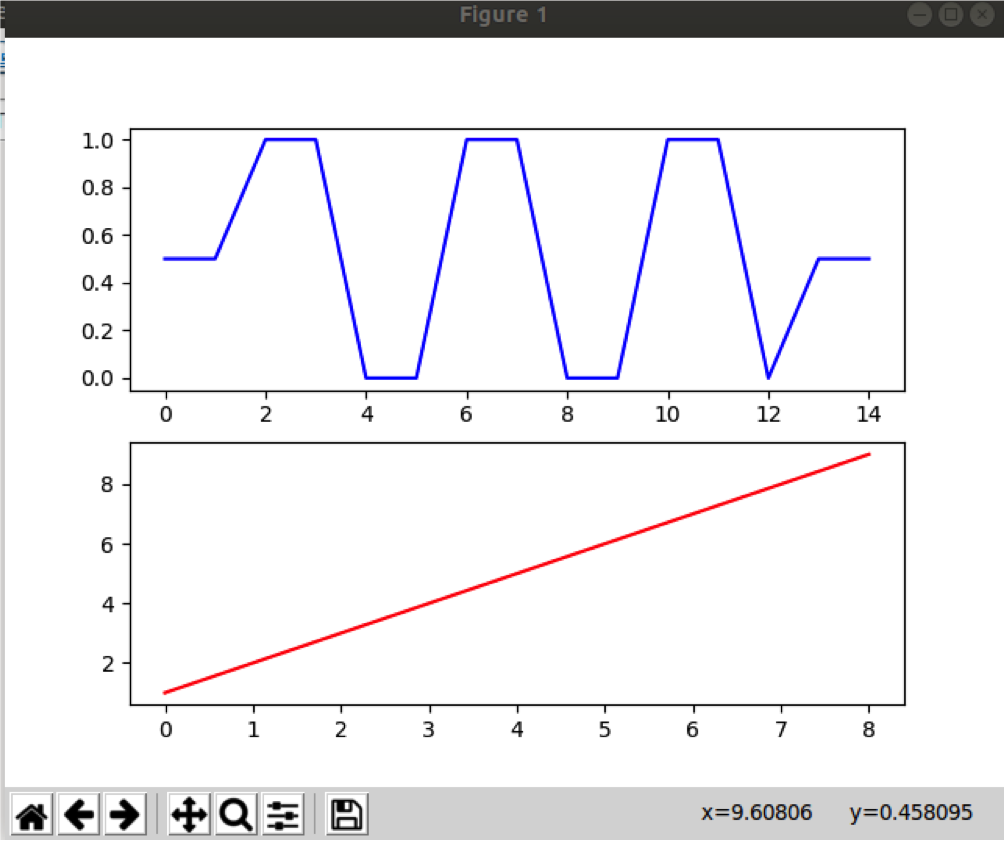Figure 2

Here we can see that two graphs are plotted separately in a subplot. The blue color indicates one, and another one is indicated by the red color. There will be no overlapping of the graph, which can confuse when the dataset is complex.

Now we have learned the basics so we can move toward some other types of graphs that Matplotlib is supporting. Matplotlib supports many types of graphs; we will see some of the essential graph types like a bar graph, stach bar graph, etc.

Bar Graph:

Unlike a line graph that shows the continuous results. The bar graph shows the height concerning other label data. The bar graph can be represented as a horizontal bar graph and a vertical bar graph. We will learn both the type of bar graph, but first, we will plot the vertical bar graph in python.

Following is the code of plotting vertical bar graph:

 code import matplotlib.pyplot as plt result = [30,20,40,10] x = [e for e in range(len(result))] fig, ax = plt.subplots() plt.bar(x, result) plt.xticks(x, ('Boys Pass', 'Boys Fail', 'Girls Pass', 'Girls Fail')) plt.show()

We first imported the Matplotlib library. As a result, we gave the data of boys passed, boys failed, girls passed, and girls failed percentage within a class. The x variable contains the list ranging from 0 to length of data set so that each data can be placed accordingly.

Then we initialize the plot and initialize the var graph in which x and result variables are provided as an argument. The x tick function takes two arguments the list of the range of data and the labels at each element of x. Then in the last, we show the data. The following graph is given as an output: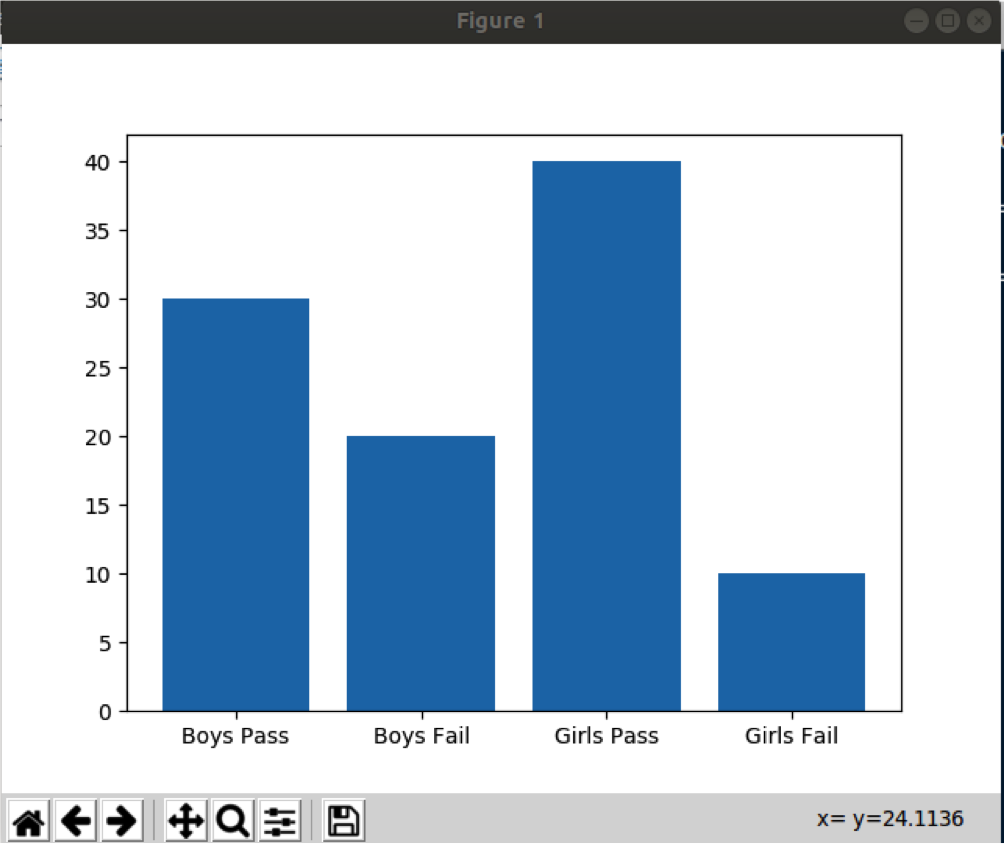Figure 3

Now to make the horizontal bar chart, we have to make the following changes:

 import matplotlib.pyplot as plt result = [30,20,40,10] x = [e for e in range(len(result))] fig, ax = plt.subplots() plt.barh(x, result) plt.yticks(x, ('Boys Pass', 'Boys Fail', 'Girls Pass', 'Girls Fail')) plt.show()

In the above code, we call the barh function instead of a bar, which initializes the horizontal bar graph. Moreover, create the label on the y-axis instead of the x-axis. The bar graph looks like the following image: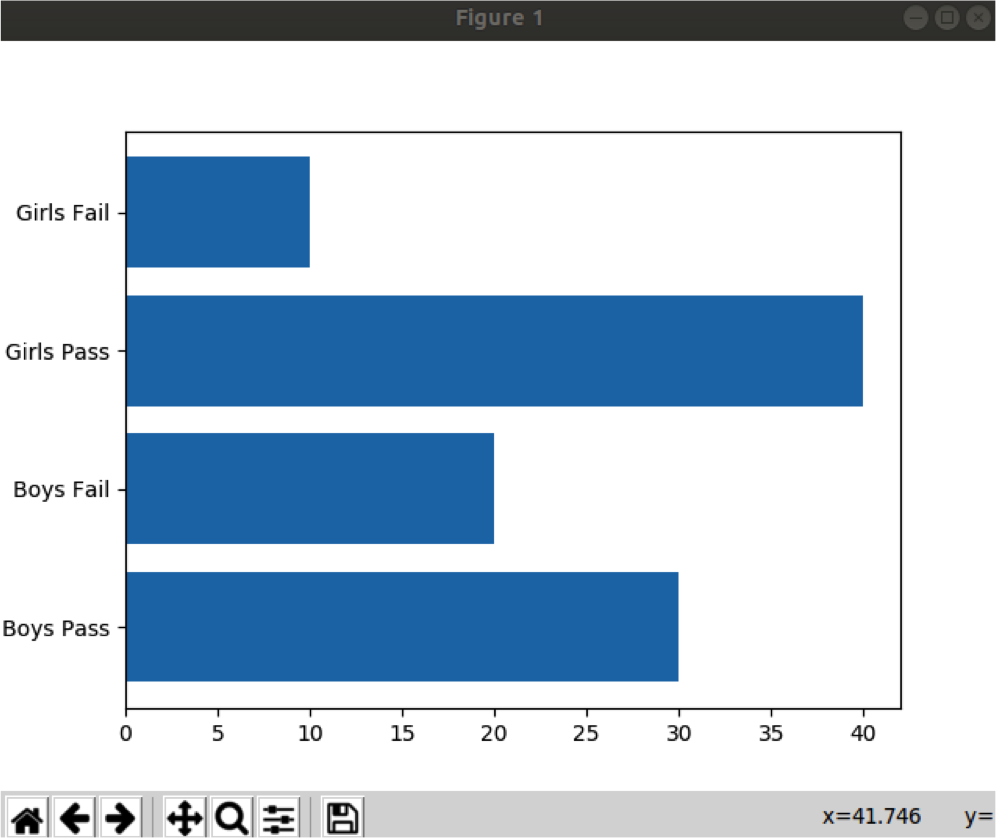Figure 4

Pie Chart:

The pie chart is another famous chart family. In a pie chart, we distribute the data in a circular 360 degree. The advantage of using the pie chart is we can see even the minute value with respect to the other value. It can give a clear visualization of the data set. Following is the code of a pie chart:

 import matplotlib.pyplot as plt   labels = ['Trumps Lover', 'Trumps Hater', 'Neutral about Trump'] percentage = [22.2, 40.8, 39] colors = ['lightgreen', 'red', 'lightblue'] p, texts = plt.pie(percentage, colors=colors, startangle=90) plt.legend(p, labels, loc="best") plt.axis('equal') plt.tight_layout() plt.show()

First, we assign the labels and then provide the data within a list. Here we have three primary data the positive data, the negative data, and the neutral data. The data that is given in the list is the percentage that will equally be distributed in a pie chart of 360 degrees. Then we have assigned the color to each data point.

The lovers are assigned a light green color, hater with red color, and neutral are given color blue. Then we initialize the pie chart and give color and percentage data as an argument. To identify each data point, we call the function legend that will show each data and what it is representing.

The tight layout function makes the pie chart tightly packed, and there will be no distance between two data points. In last we show the pie chart which looks like this: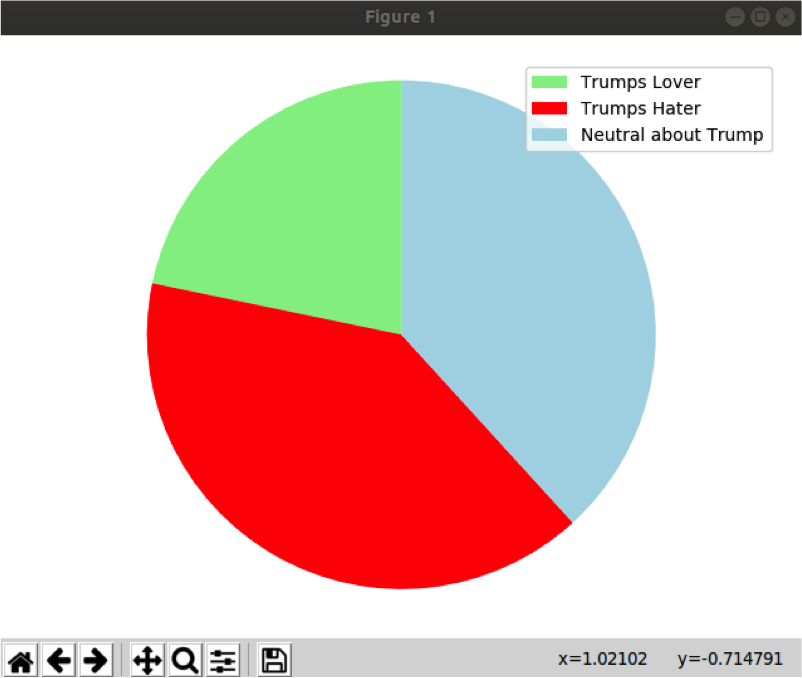Figure 5